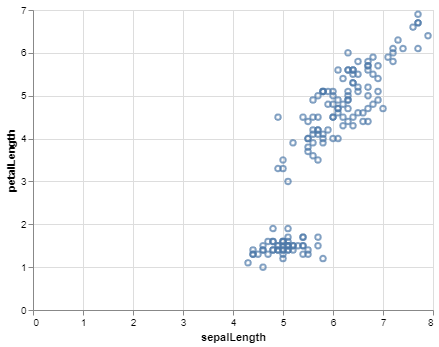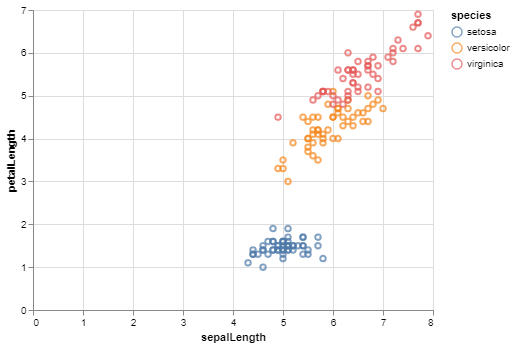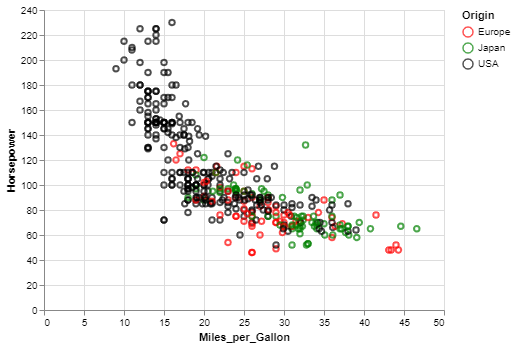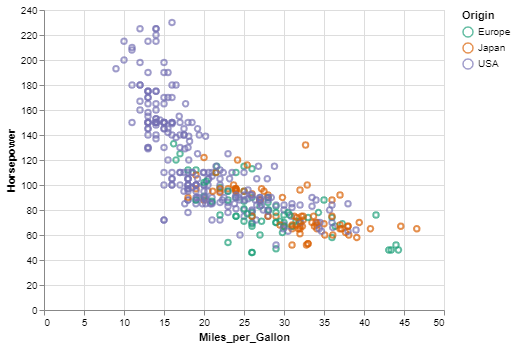Related Articles

# How To Color a Scatter Plot by a Variable in Altair?

• Last Updated : 12 Nov, 2020

Altair is a simple and easy to use statistical visualization library for python. It provides many types of visualizations ranging from simple bar charts to compound visualizations like box plots. Scatter Plot is one of the most useful visualizations in the Altair library for bivariate analysis and finding relationships between two data columns in a data set.

## Getting Started

Sometimes a simple scatter plot is not enough to gauge the relationships between the variables in a data set. A better visualization would be a plot between two quantitative variables/data columns with respect to a third variable. This third variable is almost always a nominal or categorical variable. We can color the data points in the scatter plot using this third variable. Coloring the scatter plot will help us to recognize which data point corresponds to which category of the third variable.

To color to scatter plot, the user simply has to map a nominal variable from the dataset to the color encoding.

Let us understand the importance of scatter plot coloring using an example:

The Iris dataset is one of the most popular datasets used in Data Science and is available in most dataset libraries. The dataset documents the iris flowers across three species. The data columns available in the dataset are sepalLength, sepalWidth, petalLength, petalWidth and species. First, we will visualize this dataset using a simple scatter plot and then see what can be achieved by coloring this scatter plot.

To make a simple scatter plot, we use the iris dataset from the Vega_datasets library and pass it to the Chart object and use the mark_point() method. Then, we map the x and y-axis encoding to be sepalLength and petalLength variables.

The simple scatter plot using iris without coloring:

## Python3

 `# Python3 program to illustrate``# How to color a Scatter Plot``# using altair`` ` `# Importing altair and vega_datasets library``import` `altair as alt``from` `vega_datasets ``import` `data`` ` `# Selecting the iris dataset``iris ``=` `data.iris()`` ` `# Making the Scatter Plot``alt.Chart(iris).mark_point().encode(``  ``# Map the sepalLength to x-axis``    ``x ``=` `'sepalLength'``,``  ``# Map the petalLength to y-axis``    ``y ``=` `'petalLength'``,``)`

Output:Scatter Plot for the Iris dataset  without coloring

As you can see, we can infer from this scatter plot that one group of points is linearly separable from the other group but we can’t see which data points correspond to which species and what types of relationships are present. To make this plot more informative, we will color this scatter plot using the species variable.

Code:

## Python3

 `# Python3 program to illustrate``# How to color a Scatter Plot``# using altair`` ` `# Importing altair and vega_datasets library``import` `altair as alt``from` `vega_datasets ``import` `data`` ` `# Selecting the iris dataset``iris ``=` `data.iris()`` ` `# Making the Scatter Plot``alt.Chart(iris).mark_point().encode(``  ``# Map the sepalLength to x-axis``    ``x ``=` `'sepalLength'``,``  ``# Map the petalLength to y-axis``    ``y ``=` `'petalLength'``,``  ``# Coloring the Scatter Plot``  ``# Map the species to color``    ``color ``=` `'species'``)`

Output:Scatter Plot for the Iris dataset  with coloring

Altair automatically generates the legend specifying which color represents which category of the color variable. On seeing the colored data points, we can infer that the setosa species has long sepals but short petals. The versicolor species have almost equal and medium-sized petals and sepals whereas the virginica species also have almost equal but large-sized petals and sepals.

As you can see, we can extract more information by coloring a scatter plot.

## Customizing Colors

If you don’t like the colors chosen by Altair for your scatter plot, you can customize the colors. The default colors can be changed using the scale argument of the Color class, By passing the Scale class to the scale argument. The available customizations are:

1. Custom mapping of colors to discrete values: For custom mapping, we use domain and range parameters of the Scale and pass list for values and colors resp.
2. Color Schemes: There are many color schemes given by the Vega project. If you like dark colors, you can use the ‘dark2’ scheme and if there are more than 10 categories you can use the ‘category20’ scheme.

Example 1: Custom mapping of colors to discrete values:

## Python3

 `# Python3 program to illustrate``# How to do custom mapping``# of colors to discrete values``# for scatter plot coloring``# using altair`` ` `# Importing altair and vega_datasets library``import` `altair as alt``from` `vega_datasets ``import` `data`` ` `# Selecting the cars dataset``cars ``=` `data.cars()`` ` `# Making two lists for``# values and colors resp.``dom ``=` `[``'Europe'``, ``'Japan'``, ``'USA'``]``rng ``=` `[``'red'``, ``'green'``, ``'black'``]`` ` `# Making the Scatter Plot``alt.Chart(cars).mark_point().encode(``   ` `    ``# Map Miles_per_Gallon to x-axis``    ``x``=``'Miles_per_Gallon'``,``     ` `    ``# Map the Horsepower to y-axis``    ``y``=``'Horsepower'``,``     ` `    ``# Coloring the Scatter Plot``    ``# using Origin variable and``    ``# custom colors``    ``color``=``alt.Color(``'Origin'``, scale``=``alt.``                    ``Scale(domain``=``dom, ``range``=``rng))``)`

Output:Scatter Plot for the cars dataset using custom mapping of colors and values

Example 2(Color Schemes):

## Python3

 `# Python3 program to illustrate``# How to select color schemes``# for scatter plot coloring``# using altair`` ` `# Importing altair and vega_datasets library``import` `altair as alt``from` `vega_datasets ``import` `data`` ` `# Selecting the cars dataset``cars ``=` `data.cars()`` ` `# Making the Scatter Plot``alt.Chart(cars).mark_point().encode(``   ` `    ``# Map Miles_per_Gallon to x-axis``    ``x``=``'Miles_per_Gallon'``,``     ` `    ``# Map the Horsepower to y-axis``    ``y``=``'Horsepower'``,``     ` `    ``# Coloring the Scatter Plot``    ``# using Origin variable and``    ``# color scheme``    ``color ``=` `alt.Color(``'Origin'``, scale``=``alt.``                      ``Scale(scheme ``=` `'dark2'``))``)`

Output:Scatter Plot for the cars dataset  using color scheme

Attention geek! Strengthen your foundations with the Python Programming Foundation Course and learn the basics.

To begin with, your interview preparations Enhance your Data Structures concepts with the Python DS Course. And to begin with your Machine Learning Journey, join the Machine Learning – Basic Level Course

My Personal Notes arrow_drop_up